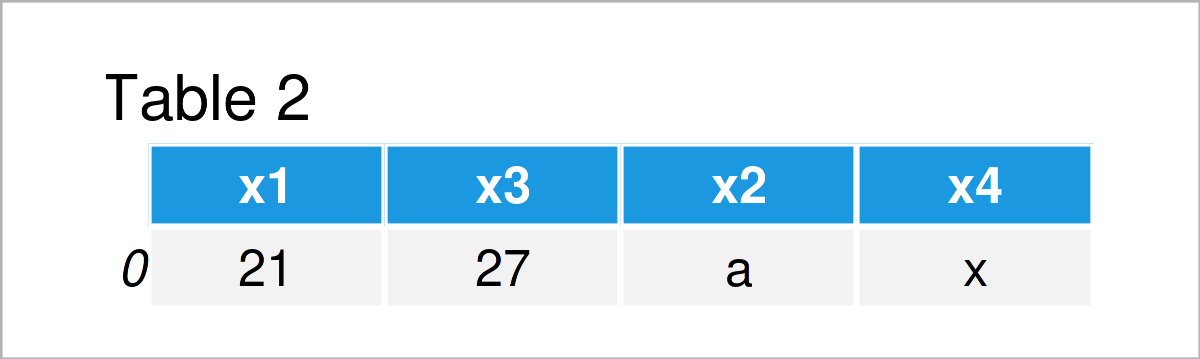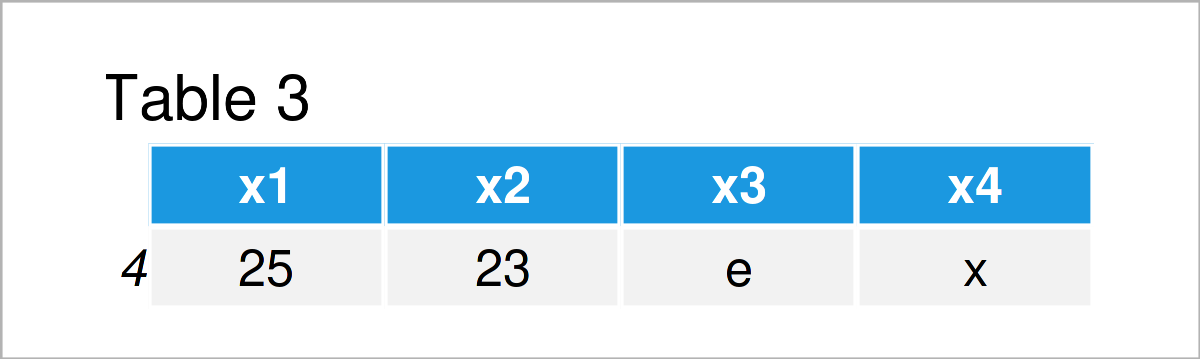# Access Index of Last Element in pandas DataFrame in Python (4 Examples)

In this tutorial, I’ll illustrate how to get access to the last element of a pandas DataFrame in the Python programming language.

The tutorial consists of the following contents:

Let’s dive right into the examples…

## Example Data & Libraries

First, we have to import the pandas library:

`import pandas as pd                                         # Load pandas library`

As a next step, we’ll also need to construct some data that we can use in the examples later on:

```data = pd.DataFrame({'x1':range(21, 26),                    # Create pandas DataFrame
'x2':range(27, 22, - 1),
'x3':['a', 'b', 'c', 'd', 'e'],
'x4':['x', 'z', 'y', 'z', 'x']})
print(data)                                                 # Print pandas DataFrame```Table 1 illustrates that our example DataFrame consists of five rows and four columns.

## Example 1: Extract First Row from pandas DataFrame

In Example 1, I’ll illustrate how to access the first row of a pandas DataFrame in Python.

To achieve this, we can use the iloc indexer as shown below:

```data_first_row = data.iloc[:1, :]                           # Using iloc indexer
print(data_first_row)                                       # Print first row```Table 2 shows the output of the previous Python code – A pandas DataFrame with only the first row of our input data set.

## Example 2: Extract First Element of Column in pandas DataFrame

In Example 2, I’ll illustrate how to access the index of the first element of a particular variable in a pandas DataFrame.

We can do this by selecting a specific column (i.e. x3) and the index position 0 within the iloc indexer:

```column_first_element = data['x3'].iloc                   # Using iloc indexer
print(column_first_element)                                 # Print first element of column
# a```

The previous Python code has returned the character “a”, i.e. the first element of the column x3.

## Example 3: Extract Last Row from pandas DataFrame

This example explains how to get the last row of a pandas DataFrame.

Once again, we can use the iloc indexer for this task. However, this time, we also have to identify the number of rows in our data set using the len function and the index attribute:

```data_last_row = data.iloc[len(data.index) - 1:, :]          # Using iloc indexer
print(data_last_row)                                        # Print last row```In Table 3 you can see that we have created another one-line data set containing the last row of our input DataFrame.

## Example 4: Extract Last Element of Column in pandas DataFrame

This example shows how to access the last element of a certain pandas DataFrame column.

For this task, we can use the iloc indexer as shown below:

```column_last_element = data['x3'].iloc[len(data.index) - 1]  # Using iloc indexer
print(column_last_element)                                  # Print last element of column
# e```

The last data cell in the column x3 contains the character e.

## Video, Further Resources & Summary

I have recently published a video on my YouTube channel, which illustrates the Python syntax of this tutorial. Please find the video below:

Please accept YouTube cookies to play this video. By accepting you will be accessing content from YouTube, a service provided by an external third party.If you accept this notice, your choice will be saved and the page will refresh.

In addition, you may want to have a look at the related Python programming articles on my website:

This article has illustrated how to select the head and tail of a pandas DataFrame in the Python programming language. In case you have additional questions or comments, don’t hesitate to let me know in the comments.

Subscribe to the Statistics Globe Newsletter